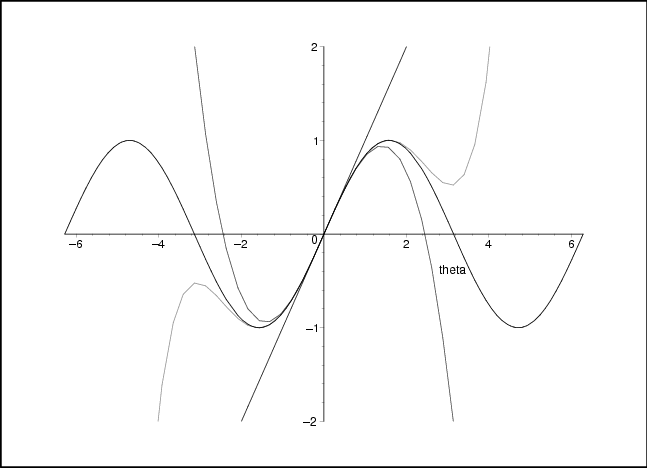## Section7.3Visualization of Power Series Approximations

In the activities in this section you have the opportunity to explore what it means to approximate a given function with a power series expansion, truncated at different orders.

### Activity7.3.1.Visualization of Power Series Approximations.

In the Sage code below or this Mathematica notebook 1 , you will begin with the function $$f(\theta)=\sin\theta\text{.}$$ Look at the first few terms of its power series. Using the coefficients that you found in Activity 7.2.2, you can plot both the function $$\sin\theta$$ and its various approximations found by truncating the power series, first after one non-zero term, then after two non-zero terms, etc. Write down as many features as you can of these approximations. In particular, answer the following questions:

1. For what values of $$\theta$$ is the approximation a “good” one? What is the definition of “good”?

2. Does the range of good values of $$\theta$$ depend on the order of the approximation? If so, how?

3. A function $$f(z)$$ is called symmetric if the graph of the function for negative values of its argument is the mirror reflection (with the vertical axis acting as mirror) of the graph of the function for positive values of its argument, i.e. if $$f(-z)=f(z)\text{.}$$ Similarly, a function is called antisymmetric if the graph of the function for negative values of its argument is the negative of the mirror reflection (with the vertical axis acting as mirror) of the graph of the function for positive values of its argument, i.e. if $$f(-z)=-f(z)\text{.}$$ Is the function $$f(z)$$ symmetric, antisymmetric, or neither? What about the various terms in the power series expansion?

Notice that if you truncate a power series, then you are looking at a polynomial whose dominant term (i.e. highest power) is the highest term that you kept in the power series. Since $$\sin\theta$$ is bounded for large $$\theta$$ and a polynomial in $$\theta$$ is not bounded, the first few terms of the power series will be a terrible approximation for the function if $$|\theta|$$ is large enough. However, as you keep more and more terms in the series, the truncated series is a better and better approximation for the function for a larger and larger range of values of $$\theta\text{.}$$

This computer algebra worksheet began by approximating $$\sin\theta$$ by $$\theta$$ near the origin —. This small angle approximation may be familiar to you from your study of the motion of a pendulum. Higher order terms add more higher order polynomials and therefore add more “bumps” to the curve; the graph of $$\sin\theta$$ has infinitely many bumps. It is remarkable that the power series for $$\sin\theta$$ converges for all values of $$\theta\text{.}$$

You can see from the graphs that all even positive integer powers of $$z$$ are symmetric; all odd positive integer powers are antisymmetric. Since the function $$f(\theta)=\sin\theta$$ is an odd function of $$\theta\text{,}$$ only the odd powers of $$\theta$$ appear in the power series expansion. You can see this antisymmetry in Figure Figure 7.3.1.

The Sage code below allows you to plot both a function $$f(x)$$ (entered at line 2) and your choice of polynomial approximation $$p(x)$$ (entered at line 3). The defaults are $$f(x)=\sin{x}$$ and $$p(x)=x$$ (the first-order term). You can change these defaults to look at any reasonable function and any order of approximation.

x=var('x')
f(x)=sin(x)
p(x)=x
F=plot(f(x),(x,-5,5))
P=plot(p(x),(x,-5,5),ymin=-2,ymax=2,color=Color('purple'))
F+P


See Figure 7.3.1.Figure 7.3.1. The function $$\sin\theta$$ and three approximations formed from truncating the power series after the first, second, and third non-zero terms (i.e. at the first, third, and fifth order.)

### Activity7.3.2.Power Series Expansions around Points Other than $$0$$.

You can (and should) also alter the same Sage or Mathematica code to make a power series approximation that fits a function well somewhere other than at the origin by writing the power series expanded “around” some other point such as $$\theta=\frac{\pi}{6}\text{.}$$

math/vfpowerapprox.nb